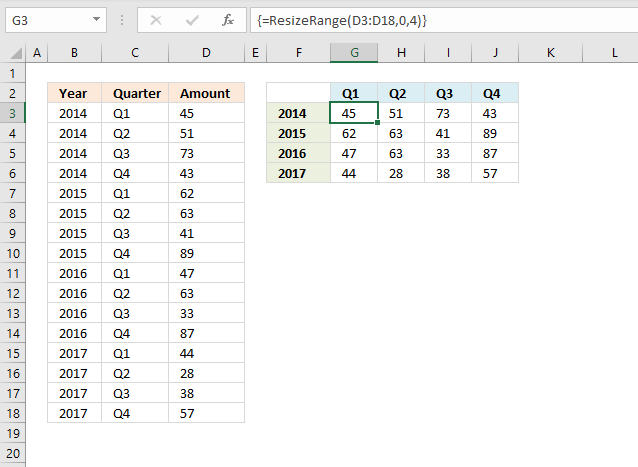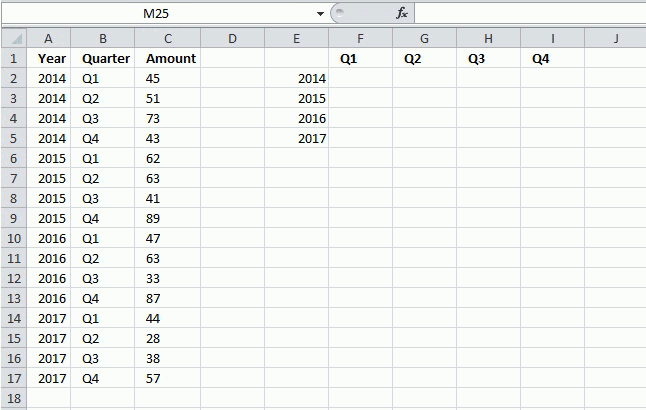Author: Oscar Cronquist Article last updated on May 25, 2021The User Defined Function (UDF) demonstrated in this article, resizes a given range to columns or rows you specify. This article Rearrange cells in a cell range to vertically distributed values can be useful to you if you want to do the opposite.

The image above shows the UDF entered in cell range G3:J6 as an array formula, it takes the values in column D and rearranges them into four columns and as many rows as needed. This article shows how to do the same using only formulas: Rearrange values using formulas

## 1. ResizeRange functionThe first argument is the range, the second argument is how many columns you want and the third argument is how many rows you want in your new range.

### UDF Syntax

=ResizeRange(rangerowscolumns)

### Arguments

range - cell range you want to resize

rows - the number of rows you want, leave it to 0 (zero) if you want the udf to calculate the number of rows needed

columns - the number of columns you want, leave it to 0 (zero) if you want the udf to calculate the number of columns needed.

The picture above shows values in column c, C2:C17 being resized into a range of 4 columns. The User Defined Function calculates the number of rows that are needed, to do that use 0 (zero) as the  row argument:

=ResizeRange(C2:C1704)

If you want 2 rows and as many columns as needed the UDF becomes:

=ResizeRange(C2:C1720)

## 2. VBA Code

```'Name User Defined Function and dimension parameters
Function ResizeRange(rng As Range, r As Single, c As Single)

'Dimension variables and declare their data types
Dim rngV As Variant
Dim tbl() As Variant
Dim Value As Variant

'Save values from range object rng to array variable rngV
rngV = rng.Value

'If ... Then ... Else ... Endif statement
If r = 0 Then

'Count cells in range object rng and divide by value in variable c, then round up to a whole number and save to variable r
r = Application.WorksheetFunction.RoundUp(rng.Cells.CountLarge / c, 0)

'Continue here if variable c is 0 (zero)
ElseIf c = 0 Then

'Count cells in range object rng and divide by value in variable r, then round up to a whole number and save to variable c
c = Application.WorksheetFunction.RoundUp(rng.Cells.CountLarge / r, 0)
End If

'Redimension array variable tbl based on variables c and r
ReDim tbl(1 To r, 1 To c)

'Save 1 to variable r
r = 1

'Save 0 (zero) to variable c
c = 0

'Transpose values in variable rngV and save to variable rngV
rngV = Application.Transpose(rngV)

'For each statement
For Each Value In rngV

'If .. Endif statement
'Check if c is equal to the number of columns in array variable tbl
If c = UBound(tbl, 2) Then

'Add 1 to the value stored in array variable r and save to variable r
r = r + 1

'Save 0 (zero) to variable c
c = 0
End If

'Add 1 to the value stored in array variable c and save to variable c
c = c + 1

'Save value in variable Value to array variable tbl
tbl(r, c) = Value

'Continue with next value
Next Value

'Return values to worksheet
ResizeRange = tbl
End Function
```

## 3. Where do I put the code?

Copy the code above to a module:
How to insert a module to a workbook.

Go back to Excel from VB Editor.

Select a cell range, type the udf and it's arguments. See animated picture at the beginning of this post.

Create an array formula, here are the details if you don't know how to.

1. Press and hold CTRL + SHIFT simultaneously
2. Press Enter
3. Release all keys

If you did it right the formula now has a curly bracket before and after. Like this {=ResizeRange(C2:C1720)}. Don't enter these characters yourself.

## 4. Explaining the User Defined Function (UDF)

Function name and arguments

A user defined function always starts with "Function" and then a name. This udf has a three arguments. Variable rng is a range object, r and c are declared as Single. Read more about Defining data types.

Function ResizeRange(rng As Range, r As Single, c As Single)

Declaring variables

Value, tbl and rngV are all variants. tbl has two parenthesis meaning it is an array. Read more about Defining data types.

Dim rngV As Variant
Dim tbl() As Variant
Dim Value As Variant

Convert values from rng (range object) to rngV (variant array)

This speeds up the function considerably if you are working with large cell ranges. Excel copies all the values from the sheet and puts them in memory (array).

rngV = rng.Value

If ... then... ElseIf ... End If

Checks if r equals to 0 (zero) and if true then calculates needed rows. Checks also if c equals to 0 (zero) and then if true calculates needed columns.

If r = 0 Then
r = Application.WorksheetFunction.RoundUp(rng.Cells.CountLarge / c, 0)
ElseIf c = 0 Then
c = Application.WorksheetFunction.RoundUp(rng.Cells.CountLarge / r, 0)
End If

Build array

Now we know how many columns and rows we need to build the array. ReDim changes the array size.

ReDim tbl(1 To r, 1 To c)

Use variables r and c to save values in array

I am reusing these variables to help me know where to put each rngV value in tbl array. r is equal to 1 and c is equal to 0 (zero).

r = 1
c = 0

Transpose array

An array with 2 rows and 4 columns becomes an array with 4 rows and 2 columns, read more about transposing an array or range.

rngV = Application.Transpose(rngV)

For ... Next statement

Repeats a group of statements a specified number of times.

For Each Value In rngV

Next Value

If ... then... End If

Checks if c is equal to the number of columns in tbl array then, if true, adds 1 to r and c is equal to 0 (zero)

If c = UBound(tbl, 2) Then
r = r + 1
c = 0
End If

c is equal to c + 1

c = c + 1

Save value to tbl array

r is the row number and c is the column number in tbl array.

tbl(r, c) = Value

Return tbl array values to function

The ResizeRange returns an array of values stored in tbl.

ResizeRange = tbl

End a udf

A function procedure ends with this statement.

End Function

### Get the Excel fileChange-number-of-rows-and-columns-in-a-rangev2.xlsm